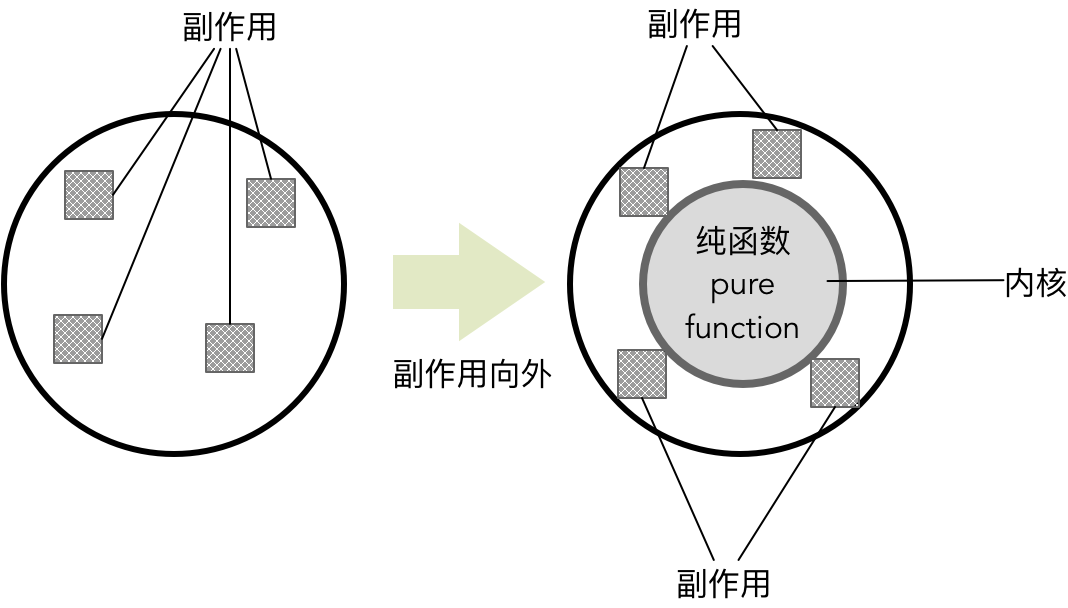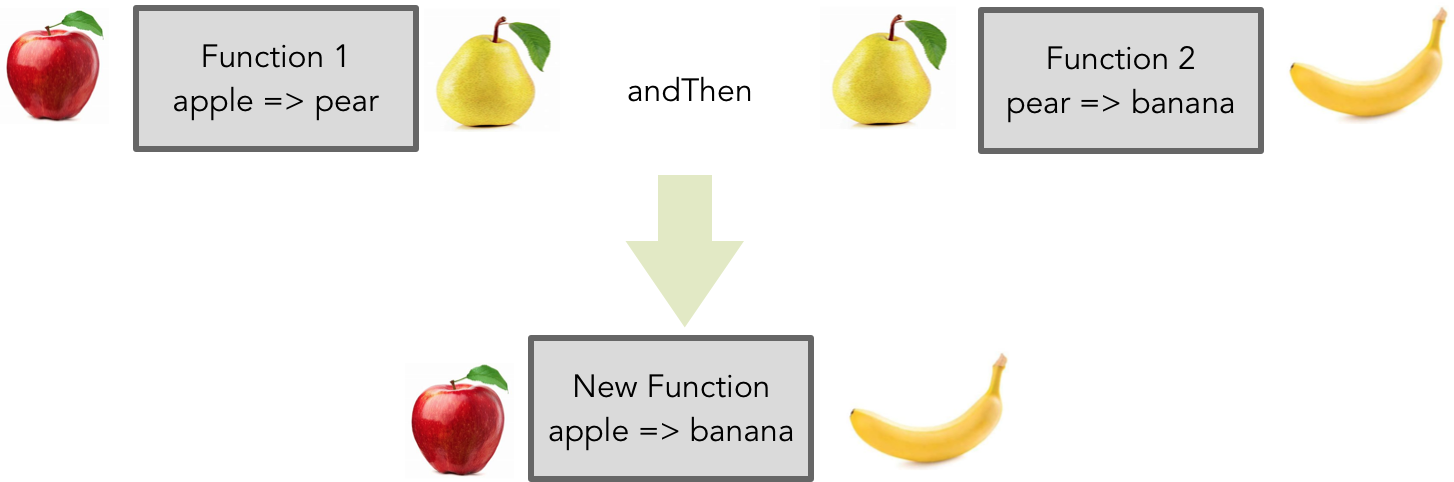054 领域模型与函数范式

函数范式

REA 的 Ken Scambler 认为函数范式的主要特征为：模块化（Modularity）、抽象化（Abstraction）和可组合（Composability），这三个特征可以帮助我们编写简单的程序。

代数数据类型

sealed trait TimeUnit

case object Days extends TimeUnit
case object Hours extends TimeUnit
case object Minutes extends TimeUnit
case object Seconds extends TimeUnit
case object MilliSeconds extends TimeUnit
case object MicroSeconds extends TimeUnit
case object NanoSeconds extends TimeUnit

type TimeUnit = Days + Hours + Minutes + Seconds + MilliSeconds + MicroSeconds + NanoSeconds

case class Employee(number: String, name: String, email: String, onboardingDate: Date)

type Employee = (String, String, String, Date)

type Employee = String * String * String * Date

sealed trait Currency
case object RMB extends Currency
case object USD extends Currency
case object EUR extends Currency

case class Balance(amount: BigDecimal, currency: Currency)

sealed trait Account {
def number: String
def name: String
}

case class SavingsAccount(number: String, name: String, dateOfOpening: Date) extends Account
case class BilledAccount(number: String, name: String, dateOfOpening: Date, balance: Balance) extends Account

val today = Calendar.getInstance.getTime
val balance = Balance(10.0, RMB)
val account = BilledAccount("980130111110043", "Bruce Zhang", today, balance)

// Amount 是一个积类型，Currency 则为前面定义的和类型
calse class Amount(value: BigDecimal, currency: Currency) {
// 实现了运算符重载，支持 Amount 的组合运算
def +(that: Amount): Amount = {
require(that.currency == currency)
Amount(value + that.value, currency)
}
def *(times: BigDecimal): Amount = {
Amount(value * times, currency)
}
}

//以下类型皆为积类型，分别体现了工作时间卡与销售凭条领域概念
case class TimeCard(startTime: Date, endTimeDate)
case class SalesReceipt(date: Date, amount: Amount)

//支付周期是一个隐藏概念，不同类型的雇员支付周期不同
case class PayrollPeriod(startDate: Date, endDate: Date)

//Income 的抽象表示成和类型与乘积类型的组合
sealed trait Income
case class WeeklySalary(feeOfHour: Amount, timeCards: List[TimeCard], payrollPeriod: PayrollPeriod) extends Income
case class MonthlySalary(salary: Amount, payrollPeriod: PayrollPeriod) extends Income
case class Commission(salary: Amount, saleReceipts: List[SalesReceipt], payrollPeriod: PayrollPeriod)

//Employee 被定义为积类型，它组合的 Income 具有不同的抽象
case class Employee(number: String, name: String, onboardingDate: Date, income: Income)

def calculateIncome(employee: Employee): Amount = employee.income match {
case WeeklySalary(fee, timeCards, _) => weeklyIncomeOf(fee, timeCards)
case MonthlySalary(salary, _) => salary
case Commision(salary, saleReceipts, _) => salary + commistionOf(saleReceipts)
}

calculateIncome() 是一个纯函数，它利用模式匹配，针对 Employee 的特定 Income 类型计算雇员的不同收入。

纯函数

• 修改一个变量
• 直接修改数据结构
• 设置一个对象的成员
• 抛出一个异常或以一个错误终止
• 打印到终端或读取用户的输入
• 读取或写入一个文件
• 在屏幕上绘画

def parse(rosterPath: String): List[Email] = {
lines.filter(containsValidEmail(_)).map(toEmail(_))
}

List(Email("[email protected]"), Email("[email protected]"))

// 调用解析方法
send(parse("roster.txt"))

// 直接调用解析结果
send(List(Email("[email protected]"), Email("[email protected]")))

def parse(content: List[String]): List[Emial] =
content.filter(containsValidEmail(_)).map(toEmail(_))

send(parse(List("liubei, [email protected]", "noname", "guanyu, [email protected]")))

send(List(Email("[email protected]"), Email("[email protected]")))def add1(x: Int):Int => x + 1

def add1(x: Int): Int => x match {
case 0 => 1
case 1 => 2
case 2 => 3
case 3 => 4
// ...
}sealed trait Fruit {
def weight: Int
}
case class Apple(weight: Int) extends Fruit
case class Pear(weight: Int) extends Fruit
case class Banana(weight: Int) extends Fruit

val appleToPear: Apple => Pear = apple => Pear(apple.weight)
val pearToBanana: Pear => Banana = pear => Banana(pear.weight)

// 使用组合
val appleToBanana = appleToPear andThen pearToBanana

scala> val appleToBanana = appleToPear andThen pearToBanana
appleToBanana: Apple => Banana = <function1>

scala> appleToBanana(Apple(15))
res0: Banana = Banana(15)

scala> val l = List("scala", "java", "python", "go")

l: List[String] = List(scala, java, python, go)

scala> l.map(lang => lang.toCharArray)
res7: List[Array[Char]] = List(Array(s, c, a, l, a), Array(j, a, v, a), Array(p, y, t, h, o, n), Array(g, o))

map 函数完成了从 List[String] 到 List[Array[Char]] 的转换。对同一个列表执行相同的转换函数，但调用 flatMap 函数：

scala> l.flatMap(lang => lang.toCharArray)

res6: List[Char] = List(s, c, a, l, a, j, a, v, a, p, y, t, h, o, n, g, o)

flatMap 函数将字符串转换为字符数组后，还执行了一次拍平操作，完成了 List[String] 到 List[Char] 的转换。

class M[A](value: A) {
private def unit[B] (value : B) = new M(value)
def map[B](f: A => B) : M[B] = flatMap {x => unit(f(x))}
def flatMap[B](f: A => M[B]) : M[B] = ...
}

flatMap、map 和 filter 往往可以组合起来，实现更加复杂的针对 Monad 的操作。一旦操作变得复杂，这种组合操作的可读性就会降低。例如，我们将两个同等大小列表中的元素项相乘，使用 flatMap 与 map 的代码为：

val ns = List(1, 2)
val os = List(4, 5)
val qs = ns.flatMap(n => os.map(o => n * o))

val qs = for {
n <- ns
o <- os
} yield n * o

// 积类型
case class Order(id: OrderId, customerId: CustomerId, desc: String, totalPrice: Amount, discount: Amount, shippingFee: Amount, orderItems: List[OrderItem])

// 以下是验证订单的行为，皆为原子的纯函数，并返回 scalaz 定义的 Validation Monad
val validateOrder : Order => Validation[Order, Boolean] = order =>
if (order.orderItems isEmpty) Failure(s"Validation failed for order \$order.id")
else Success(true)

val checkCustomerStatus: Order => Validation[Order, Boolean] = order =>
Success(true)

val checkInventory: Order => Validation[Order, Boolean] = order =>
Success(true)

// 以下定义了计算订单的行为，皆为原子的纯函数
val calculateTotalPrice: Order => Order = order =>
val total = totalPriceOf(order)
order.copy(totalPrice = total)

val calculateDiscount: Order => Order = order =>
order.copy(discount = discountOf(order))

val calculateShippingFee: Order => Order = order =>
order.copy(shippingFee = shippingFeeOf(order))

val order = ...

// 组合验证逻辑
// 注意返回的 orderValidated 也是一个 Validation Monad
val orderValidated = for {
_ <- validateOrder(order)
_ <- checkCustomerStatus(order)
c <- checkInventory(order)
} yield c

if (orderValidated.isSuccess) {
// 组合计算逻辑，返回了一个组合后的函数
val calculate = calculateTotalPrice andThen calculateDiscount andThen calculateShippingFee
// 返回具有订单总价、折扣与运费的订单对象
// 在计算订单的过程中，订单对象是不变的
val calculatedOrder = calculate(order)

// ...
}

函数范式与领域模型

Debasish Ghosh 总结了函数范式的基本原则，用以建立更好的领域模型：

• 利用函数组合的力量，用小函数组装成一个大函数，获得更好的组合性。
• 纯粹，领域模型的很多部分都由引用透明的表达式组成。
• 通过方程式推导，可以很容易地推导和验证领域行为。

sealed trait Event {
def eventId: EventId
def occurred: Time
}

case class AccountOpened(eventId: EventId, Account: Account, occurred: Time) extends Event

def handle(event: Event) = event match {